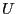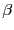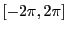Next: Analyzing univariate polynomials Up: Utilities for trigonometric equation Previous: Distance between two angles   Contents

Generalized inverse trigonometric functions

Assume that we havewhereis an interval andshould lie in an arbitrary range. The procedure Filtre_Arc_Cos allows to update the range for. It returns -1 isandare incompatible, 0 otherwise. Its syntax is:

int Filtre_Arc_Cos(INTERVAL &U,INTERVAL &beta)
A similar procedure exist for the inverse sine with

The procedure Arc_Cos_Multiple allows to determine all possible ranges for, assuming thatis restricted to a range included in::

int Arc_Cos_Multiple(INTERVAL &U,INTERVAL &beta,INTERVAL_VECTOR &BETA)
This procedure returns the number of possible ranges forand their values in BETA.

Jean-Pierre Merlet 2012-12-20